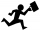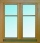# Expression of a variable from formula - examples - page 29

1. A reportMaricris can type a committee report in 5 hours. Jane helped Maricris and together they finished the report in 3 hours. How long would it take Jane to complete the report she had worked alone?
2. Rectangle 45The perimeter of a rectangle is 60cm. If the length of the rectangle is 20cm. a)find the width b)find the area.
3. GivenGiven 2x =0.125 find the value of x
4. Greg and BillGreg is 18 years old. He is 6 less than 4 times Bill's age. How old is Bill?
5. Workers 9If two workers fix two windows in an hour how many windows can 18 workers fix in the same two windows?
6. Rectangular plotThe dimensions of a rectangular plot are (x+1)m and (2x-y)m. If the sum of x and y is 3m and the perimeter of the plot is 36m. Find the area of the diagonal of the plot.

We apologize, but in this category are not a lot of examples.
Do you have an interesting mathematical example that you can't solve it? Enter it, and we can try to solve it.

To this e-mail address, we will reply solution; solved examples are also published here. Please enter e-mail correctly and check whether you don't have a full mailbox.